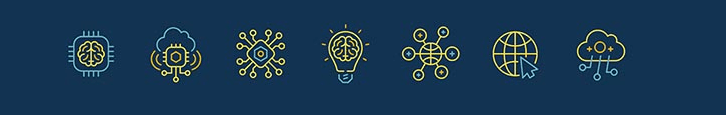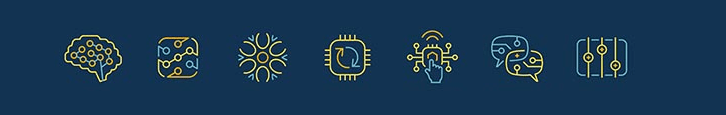DS Tutorial

# Relationship Between Variables

In Data Science, one of the most common tasks is to assess the strength of associations between two or more variables. This fundamental analysis is essential for understanding relationships, dependencies, and patterns within the data. Statistics provides a comprehensive toolkit for performing these analyses, ranging from examining the characteristics of a single variable (Univariate statistics) to exploring the relationships between pairs of variables (Bivariate statistics), and even analyzing the interactions among multiple variables (Multivariate statistics).

## Univarate Statistics

Univariate statistics involves analyzing a single variable in isolation, providing valuable insights into its central tendency, variability, and distribution. This type of analysis is often employed to understand the characteristics and patterns of a single data attribute.

## Bivariate Statistics

Bivariate statistics, on the other hand, revolves around exploring the relationships between two variables. It delves into correlation and regression analyses, enabling researchers to quantify the strength and direction of associations between pairs of variables. Bivariate statistics facilitate the identification of cause-and-effect relationships, helping to uncover how changes in one variable impact the other.

## Multivariate Statistics

Multivariate statistics delve into the interactions and relationships among three or more variables simultaneously. These analyses can be more complex and are useful when dealing with datasets where multiple variables interact to influence outcomes. Techniques like factor analysis, cluster analysis, and principal component analysis fall under the realm of multivariate statistics, aiding in identifying underlying patterns and dependencies among multiple variables.## Correlation

Correlation is a statistical technique that can perform whether and how strongly pairs of variables are related. If the two variables move in the same direction , then those variables are said to have a positive correlation. If they move in opposite directions , then they have a negative correlation.

## Correlation Coefficient

The Correlation Coefficient is a statistical measure of the strength of the linear association between the relative movements of two variables. The correlation coefficient always takes a value between -1 and 1.

1. 1 indicates a strong positive relationship.
2. -1 indicates a strong negative relationship.
3. 0 indicates no relationship at all.

## Covariance

In statistics, a Covariance refers to the measure of how two random variables will change when they are compared to each other. In other words, it defines the changes between the two variables , such that change in one variable is equal to change in another variable. Unlike the correlation coefficient , covariance is measured in units. The units are computed by multiplying the units of the two variables.

## Causation

Causation indicates a relationship between two events where one event is affected by the other. It explicitly applies to cases where action A causes outcome B i.e. there is a causal relationship between the two events. This is also referred to as cause and effect . For example: when the value of one event, or variable, increases or decreases as a result of other events, it is said there is causation .## Pearson's Correlation

Pearson correlation coefficient "r" is defined in statistics as the measurement of the strength of the linear relationship or association between two continuous variables and their association with each other. The Pearson correlation method assigns a value between - 1 and 1, where 0 is no correlation, 1 is total positive correlation, and - 1 is total negative correlation. Pearson correlations are only suitable for quantitative variables (including dichotomous variables).

## Spearman's Correlation

Spearman's correlation is a statistical measure of the strength and direction of association that exists between two variables measured on at least an ordinal scale .

### Conclusion

The application of statistics in Data Science enables researchers to unravel the intricacies of data, uncover meaningful insights, and make data-driven decisions. By using the various forms of statistical analysis, data scientists gain a comprehensive understanding of the interplay between variables, allowing them to draw valuable conclusions, build predictive models, and unearth hidden relationships that drive the behavior of the data. Embracing the diverse range of statistical techniques empowers data scientists to navigate the complexities of real-world datasets, extract valuable information, and uncover actionable knowledge from the data at hand.